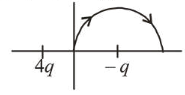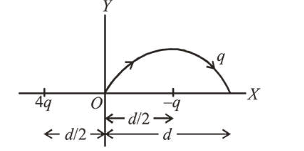# A two point charges $4 q$ and $-q$ are fixed on the $x$-axis atQuestion:

A two point charges $4 q$ and $-q$ are fixed on the $x$-axis at

$x=-\frac{d}{2}$ and $x=\frac{d}{2}$, respectively. If a third point charge

' $q$ ' is taken from the origin to $x=d$ along the semicircle as shown in the figure, the energy of the charge will:1. (1) increase by $\frac{3 q^{2}}{4 \pi \varepsilon_{0} d}$

2. (2) increase by $\frac{2 q^{2}}{3 \pi \varepsilon_{0} d}$

3. (3) decrease by $\frac{q^{2}}{4 \pi \varepsilon_{0} d}$

4. (4) decrease by $\frac{4 q^{2}}{3 \pi \varepsilon_{0} d}$

Correct Option: , 4

Solution:

(4) Change in potential energy, $\Delta u=q\left(V_{f}-V_{i}\right)$

Potential of $-q$ is same as initial and final point of the path.$\Delta u=q\left(\frac{k 4 q}{3 d / 2}-\frac{k 4 q}{d / 2}\right)=-\frac{4 q^{2}}{3 \pi \varepsilon_{0} d}$

-ve sign shows the energy of the charge is decreasing.